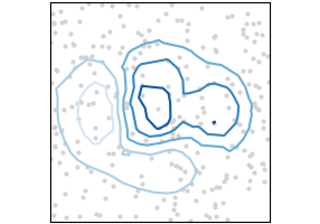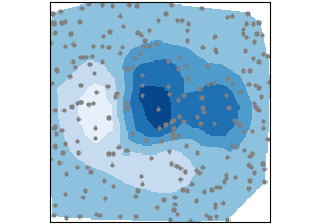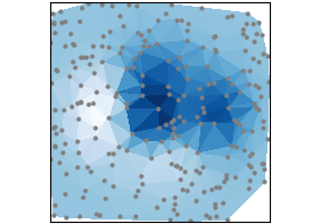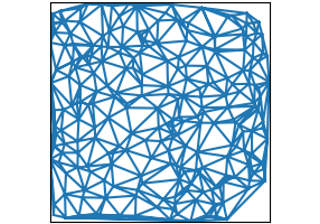# Unstructured coordinates#

Sometimes we collect data `z` at coordinates `(x,y)` and want to visualize as a contour. Instead of gridding the data and then using `contour`, we can use a triangulation algorithm and fill the triangles.tricontour(x, y, z)

tricontour(x, y, z)tricontourf(x, y, z)

tricontourf(x, y, z)tripcolor(x, y, z)

tripcolor(x, y, z)triplot(x, y)

triplot(x, y)